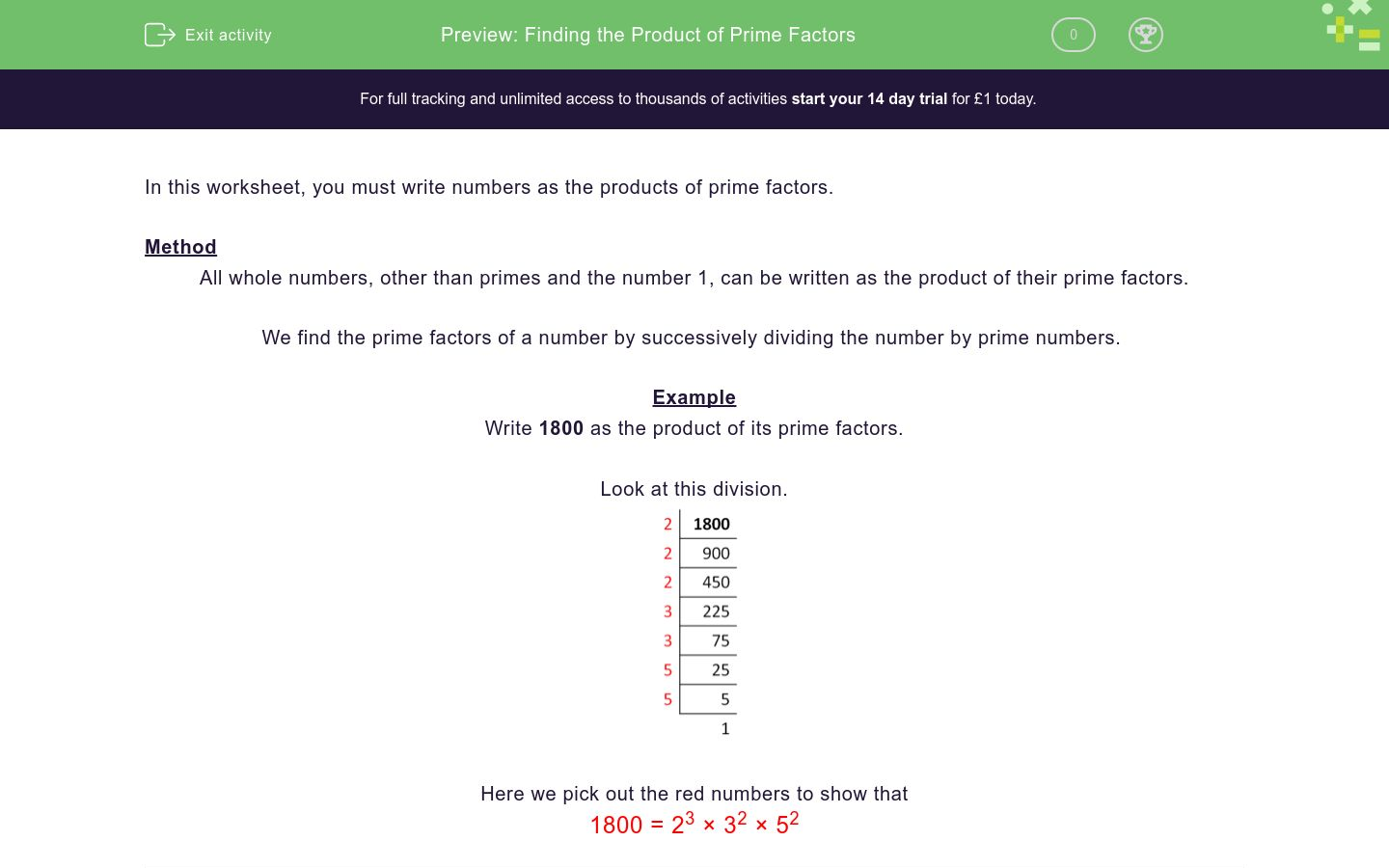# Finding the Product of Prime Factors

In this worksheet, students practise finding the product of prime factors.Key stage:  KS 3

Curriculum topic:   Number

Curriculum subtopic:   Use Concepts and Vocabulary for All Numbers

Difficulty level:### QUESTION 1 of 10

In this worksheet, you must write numbers as the products of prime factors.

Method

All whole numbers, other than primes and the number 1, can be written as the product of their prime factors.

We find the prime factors of a number by successively dividing the number by prime numbers.

Example

Write 1800 as the product of its prime factors.

Look at this division.Here we pick out the red numbers to show that

1800 = 23 × 32 × 52

Write the following number as the product of its prime factors.

3897

32 × 433

33 × 433

34 × 433

31 × 433

Write the following number as the product of its prime factors.

847

7×112

23 × 421

25 × 61

112 × 5

Write the following number as the product of its prime factors.

6540

23 × 3 × 5 × 109

22 × 3 × 5 × 71

22 × 3 × 5 × 109

32 × 3 × 5 × 109

Write the following number as the product of its prime factors.

450

2×32 ×53

2×72 ×52

2×32 ×52

101 × 45

Write the following number as the product of its prime factors.

350

2×52 ×73

2×52 ×7

2×32 ×52

351 × 10

Write the following number as the product of its prime factors.

3780

2×52 ×73

2×52 ×7

22 ×33×5×7

2×32 ×72

Write the following number as the product of its prime factors.

5733

32 ×72×13

3×52 ×7

52 ×33×5×7

11×32 ×72

Write the following number as the product of its prime factors.

8910

32 ×72×13

3×112 ×7

52 ×33×5×7

11×34 ×5×2

Write the following number as the product of its prime factors.

11907

35 ×72

3×112 ×7

72 ×33×5×7

11×74 ×5×2

Write the following number as the product of its prime factors.

29750

2×52 ×73

2×53 ×7×17

2×32 ×52

31 × 11

• Question 1

Write the following number as the product of its prime factors.

3897

32 × 433
• Question 2

Write the following number as the product of its prime factors.

847

7×112
• Question 3

Write the following number as the product of its prime factors.

6540

22 × 3 × 5 × 109
• Question 4

Write the following number as the product of its prime factors.

450

2×32 ×52
• Question 5

Write the following number as the product of its prime factors.

350

2×52 ×7
• Question 6

Write the following number as the product of its prime factors.

3780

22 ×33×5×7
• Question 7

Write the following number as the product of its prime factors.

5733

32 ×72×13
• Question 8

Write the following number as the product of its prime factors.

8910

11×34 ×5×2
• Question 9

Write the following number as the product of its prime factors.

11907

35 ×72
• Question 10

Write the following number as the product of its prime factors.

29750

2×53 ×7×17
---- OR ----

Sign up for a £1 trial so you can track and measure your child's progress on this activity.

### What is EdPlace?

We're your National Curriculum aligned online education content provider helping each child succeed in English, maths and science from year 1 to GCSE. With an EdPlace account you’ll be able to track and measure progress, helping each child achieve their best. We build confidence and attainment by personalising each child’s learning at a level that suits them.

Get started